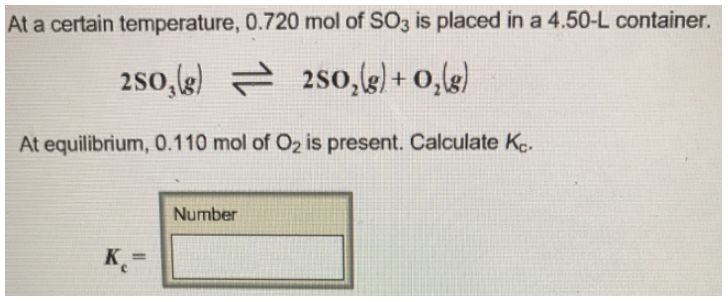# Problem: At a certain temperature, 0.720 mol of SO3 is placed in a 4.50-L container. 2SO3 (g) ⇌ 2SO2 (g) + O2 (g) At equilibrium, 0.110 mol of O2 is present. Calculate Kc.

🤓 Based on our data, we think this question is relevant for Professor Bell's class at PITT.

###### FREE Expert Solution###### Problem Details

At a certain temperature, 0.720 mol of SO3 is placed in a 4.50-L container.

2SO(g) ⇌ 2SO2 (g) + O2 (g)

At equilibrium, 0.110 mol of O2 is present. Calculate Kc